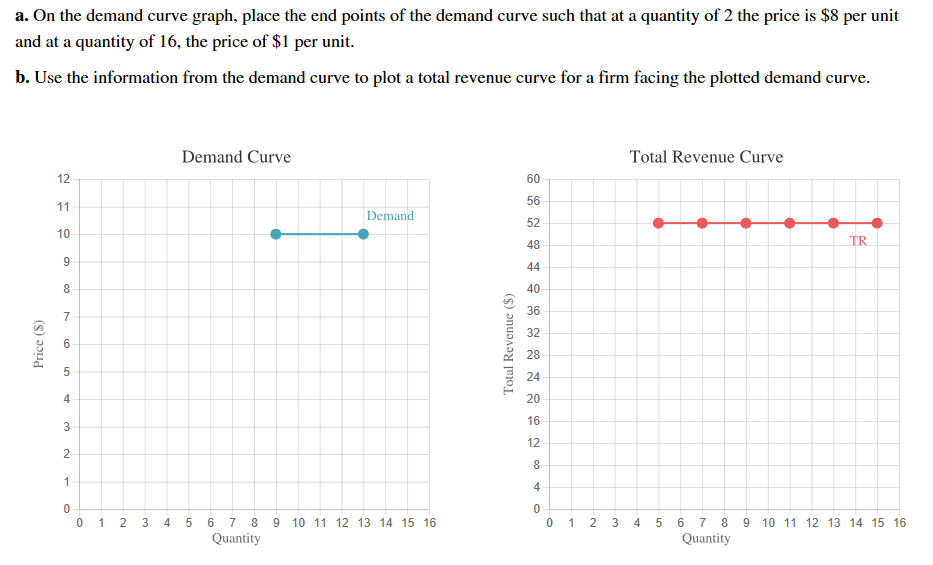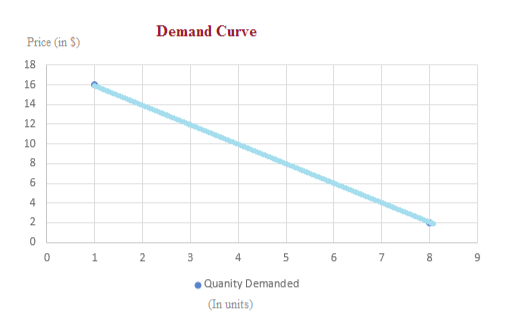a. On the demand curve graph, place the end points of the demand curve such that at a quantity of 2 the price is \$8 per unitand at a quantity of 16, the price of \$1 per unitb. Use the information from the demand curve to plot a total revenue curve for a firm facing the plotted demand curveDemand CurveTotal Revenue Curve12605611Demand5210TR48944840367326282442016122814000 1 2 30 1 2 3 4 5 6 7 8 9 10 11 12 13 14 15 164 5 6 7 810 11 12 13 14 15 16QuantityQuantityTotal Revenue (\$)coLO(s)эPrice

Questionhelp_outlineImage Transcriptionclosea. On the demand curve graph, place the end points of the demand curve such that at a quantity of 2 the price is \$8 per unit and at a quantity of 16, the price of \$1 per unit b. Use the information from the demand curve to plot a total revenue curve for a firm facing the plotted demand curve Demand Curve Total Revenue Curve 12 60 56 11 Demand 52 10 TR 48 9 44 8 40 36 7 32 6 28 24 4 20 16 12 2 8 1 4 0 0 0 1 2 3 0 1 2 3 4 5 6 7 8 9 10 11 12 13 14 15 16 4 5 6 7 8 10 11 12 13 14 15 16 Quantity Quantity Total Revenue (\$) co LO (s) э Price fullscreen
Step 1

Demand Curve: It refers to the curve that represents the relation between the demand for a commodity and its price keeping other things constant.

Total Revenue: It refers to the sum total of all the receipts from the sale of a good calculated by multiplying price and quantity of the commodity.

Step 2

(a) When the price is \$8 the quantity demanded is 2 units and at a quantity of 16 units t...help_outlineImage TranscriptioncloseDemand Curve Price (in S) 18 16 14 12 10 8 6 4 2 0 0 1 2 3 4 5 6 7 9 Quanity Demanded (In units) un fullscreen

Want to see the full answer?

See Solution

Want to see this answer and more?

Our solutions are written by experts, many with advanced degrees, and available 24/7

See Solution
Tagged in